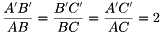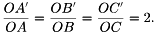## Multiplication of Integers (Practice Quiz)

#### How to Use the Applet

1.) Use the sliders to adjust the minimum and maximum values of the factors.
4.) Click the “New Set” command button to generate new sets of factors.

## Subtraction of Integers (Practice Quiz)

#### How to Use the Applet

1.) Use the sliders to adjust the minimum and maximum values of the minuends/subtrahends.
4.) Click the “New Set” command button to generate new sets of minuends/subtrahends.

## Slope and y-intercept

Move sliders m and b below to investigate the graph of y = mx + b.

Questions

1. How does the value of m and b affect the graph of y = mx + b?
2. .Without using GeoGebra, explain and justify what should be the values of m and b so that the graph passes through
• Quadrants I, II, and III
• Quadrants I, II, and IV
• Quadrants I and III only
• Quadrants III and IV only
3. Explain and justify why
• m > 0 when the graph slants to the right.
• m < 0 when the graph slants to the left.
• m = 0 when the graph is horizontal.

## Addition of Integers (Practice Quiz)

How to Use the Applet
1.) Use the sliders to adjust the minimum and maximum values of the addends.
4.) Click the “New Set” command button to generate a new sets of addends.

## The Midline Theorem

Two midpoints of a triangle are connected with a segment. Investigate.

1.) What did you observe in your investigation?

2.) What do your observations about the measures of the angles mean? How do they relate to the properties of the segments?

3.) What did you observe about the lengths of segment DE and segment BC? Was this observation always true?

4.) Based on the discussion, make conjectures about the relationship between segment AB and segment BC.

## The Pantograph

A pantograph is a mechanical linkage connected in a special manner based on parallelograms so that the movement of one pen, in tracing an image, produces identical movements in a second pen. If a line drawing is traced by the first point, an identical, enlarged or miniaturized copy will be drawn by a pen fixed to the other. The pantograph was invented by Cristoph Scheiner.

The applet above demonstrates how the pantograph works.  Move the blue point (hinge) and draw any shape.

Questions:
1.) What do you observe?
2.) What is the relationship between the blue figure and red figure?
4.) Prove that the relationship in 2 always holds.

## Pythagorean Theorem

Four right triangles with sides a, b, and hypotenuse c, are arranged to form square GHIJ. You may adjust the size of the triangles by moving point G and their shape by moving point Q. Answer questions 1 and 2 first before moving the slider.

2) What is the area of the quadrilateral in (1)?

3) Move slider R to the extreme right to show slider T. Then, move slider T to the extreme right. What type/s of quadrilateral are the two white regions? Justify your answer.

4.) What are the areas of the quadrilaterals in (3)?

5.) How does the area of the quadrilateral in (1) relate to the areas of the quadrilaterals in (3)?

6.) What equation can we form from the relationship in (4) in terms of a, b, and c?

## Copying a Segment

This applet mimics compass and straightedge construction on copying a segment. Move the slider to the right to copy segment AB.

## Task 5 – Distance from Point of Similarity

In the figure below,$\triangle ABC \sim A'B'C'$ and point$P$ is the point of similarity of the two triangles.

1.) What is the ratio of the lengths of the corresponding sides?

2.) What is the ratio of the distance from point P to the corresponding vertices?

3.) What conjecture can you make from your answer in 1 and 2? Verify your conjectures by drawing a different pair of similar triangles.

Explanation

The ratio of the corresponding sides of two similar triangles is the same as the ratio of the distance of their corresponding vertices to the center of similarity. The ratio of the corresponding sides of two similar triangles is the same as the ratio of the distance of their corresponding vertices. In the figure above, ∆ABC ~ ∆A’B’C’ soand## Task 4 – Point of Similarity

Shown below are$\triangle STU$ and$\triangle MNO$.

Questions

1.) Are the two triangles similar? Why?

2.) What is the ratio of the lengths of their corresponding sides?

3.) Use the Line tool to connect the corresponding vertices of the two triangles. Did your observations in Task 2 and Task 3 hold?

4.) Make a conjecture about the lines connecting the corresponding vertices of similar triangles.

### ’Explanation’

If two triangles are similar and their corresponding sides are parallel, then the line connecting their corresponding vertices will intersect at a point. This point is called the center of similarity or point of similarity.

In Task 3,$\triangle ABC \sim \triangle A'B'C'$ and their corresponding sides are parallel. The three lines connecting the corresponding vertices intersect point$P$ as shown above. In Task 4,$\triangle STU \sim \triangle MNO$, but their corresponding sides are not parallel, so the three lines connecting their corresponding vertices do not intersect at a point.